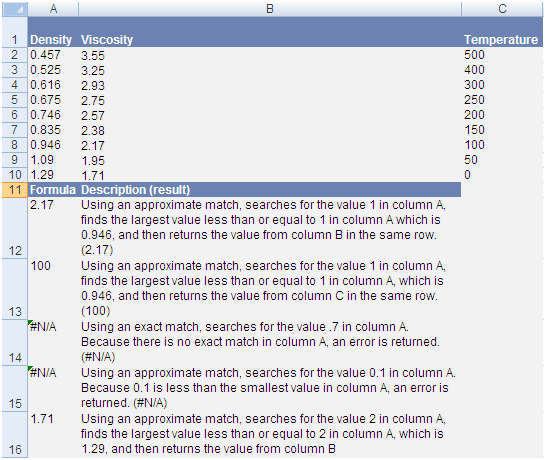# How to use Excel Vlookup example and descriptionIn Excel Vlookup function is used to search the value in array and then bring back the value form indexed column

Vlookup consist of four parts and each part is separated by comma

# Vlookup formula

VLOOKUP(lookup_value,table_array,col_index_num,range_lookup)

# Lookup_value

It is the values to be searched in first column of array if Vlookup function found the looking value then it will bring the value motioned in col_index_num otherwise it will result in #N/A error value

# Table_array

This is the set of column in which Vlookup perform its function of lookup, It can be consist of two or more columns of data. It can be Use a reference to a range or a range name. Vlookup will search the values in the first column of table_array. These values can be numbers text, numbers, logical values etc. Upper and lower case are text equivalent.

# col_index_num

Column index is column number in table_array from which you want the value to return, col_index_num
1 will bring the value from 1st column of table_array and
col_index_num
2 will bring the value from 2nd column of table_array

# range_lookup

This part repent that whatever you are looking for you wants exact match or approximate match, it can either be False or True

False means it will match the exact value

Ture mean it will match the exact but if not available then it will match it with closest value

# Vlookup example# Vlookup excel example

Copy and paste the below table in Excel sheet cell A1

 Density Viscosity Temperature 0.457 3.55 500 0.525 3.25 400 0.616 2.93 300 0.675 2.75 250 0.746 2.57 200 0.835 2.38 150 0.946 2.17 100 1.09 1.95 50 1.29 1.71 0 Formula Description (result) 2.17 Using an approximate match, searches for the value 1 in column A, finds the largest value less than or equal to 1 in column A which is 0.946, and then returns the value from column B in the same row. (2.17) 100 Using an approximate match, searches for the value 1 in column A, finds the largest value less than or equal to 1 in column A, which is 0.946, and then returns the value from column C in the same row. (100) #N/A Using an exact match, searches for the value .7 in column A. Because there is no exact match in column A, an error is returned. (#N/A) #N/A Using an approximate match, searches for the value 0.1 in column A. Because 0.1 is less than the smallest value in column A, an error is returned. (#N/A) 1.71 Using an approximate match, searches for the value 2 in column A, finds the largest value less than or equal to 2 in column A, which is 1.29, and then returns the value from column B